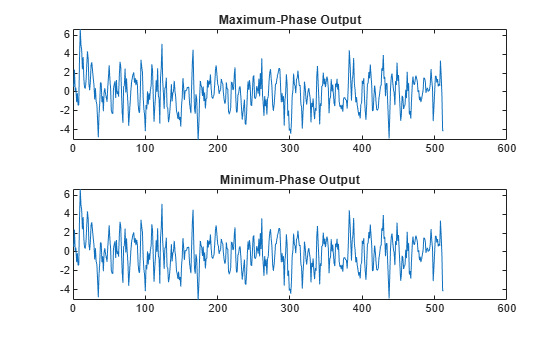latcfilt

Syntax

[f,g] = latcfilt(k,x)
[f,g] = latcfilt(k,v,x)
[f,g] = latcfilt(k,1,x)
[f,g,zf] = latcfilt(...,'ic',zi)
[f,g,zf] = latcfilt(...,dim)

Description

When filtering data, lattice coefficients can be used to represent

• FIR filters

• All-pole IIR filters

• Allpass IIR filters

• General IIR filters

[f,g] = latcfilt(k,x) filters x with the FIR lattice coefficients in the vector k. The forward lattice filter result is f and g is the backward filter result. If $|k|\le 1$, f corresponds to the minimum-phase output, and g corresponds to the maximum-phase output.

If k and x are vectors, the result is a (signal) vector. Matrix arguments are permitted under the following rules:

• If x is a matrix and k is a vector, each column of x is processed through the lattice filter specified by k.

• If x is a vector and k is a matrix, each column of k is used to filter x, and a signal matrix is returned.

• If x and k are both matrices with the same number of columns, then the ith column of k is used to filter the ith column of x. A signal matrix is returned.

[f,g] = latcfilt(k,v,x) filters x with the IIR lattice coefficients k and ladder coefficients v. Both k and v must be vectors, while x can be a signal matrix.

[f,g] = latcfilt(k,1,x) filters x with the IIR lattice specified by k, where k and x can be vectors or matrices. f is the all-pole lattice filter result and g is the allpass filter result.

[f,g,zf] = latcfilt(...,'ic',zi) accepts a length-k vector zi specifying the initial condition of the lattice states. Output zf is a length-k vector specifying the final condition of the lattice states.

[f,g,zf] = latcfilt(...,dim) filters x along the dimension dim. To specify a dim value, the FIR lattice coefficients k must be a vector and you must specify all previous input parameters in order. Use the empty vector [ ] for any parameters you do not want to specify. zf returns the final conditions in columns, regardless of the shape of x.

Examples

collapse all

Generate a signal with 512 samples of white Gaussian noise.

x = randn(512,1);

Filter the data with an FIR lattice filter. Specify the reflection coefficients so that the lattice filter is equivalent to a 3rd-order moving average filter.

[f,g] = latcfilt([1/2 1],x);

Plot the maximum- and minimum-phase outputs of the lattice filter in separate plots

subplot(2,1,1)
plot(f)
title('Maximum-Phase Output')

subplot(2,1,2)
plot(g)
title('Minimum-Phase Output')